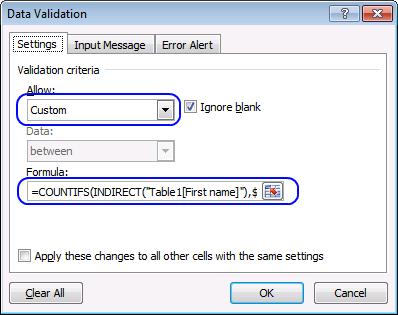Author: Oscar Cronquist Article last updated on February 27, 2018

Debra Dalgleish demonstrated last week how to block duplicate entries in excel table. I made a comment and I thought I would share it with you.

Here is an excel table with example data.

Let me show you how to prevent duplicate records in this table.

1. Select cell range B3:D8
2. Go to tab "Data" on the ribbon
3. Click "Data validation" button4. Choose Custom
5. Type in "Formula:" field:
=COUNTIFS(INDIRECT("Table1[First name]"), \$B3, INDIRECT("Table1[Last name]"), \$C3, INDIRECT("Table1[Date]"), \$D3)<=1
6. Click OK

If you enter a duplicate record, this error message appears.

### Explaining the data validation formula in row 9

Step 1 - How to use table names in excel data validation formulas

You can´t use table names in excel conditional formatting formulas or data validation formulas. However the INDIRECT function allows you to do that.

Table1[First name] -> INDIRECT("Table1[First name]")

Step 2 - Count how many times a record exists in a table

COUNTIFS(INDIRECT("Table1[First name]"), \$B9, INDIRECT("Table1[Last name]"), \$C9, INDIRECT("Table1[Date]"), \$D9)

becomes

COUNTIFS(\$B\$3:\$B\$9, \$B9, \$C\$3:\$C\$9, \$C9, \$C\$3:\$D\$9, \$D9)

becomes

COUNTIFS({"Stieg"; "Jonas"; "Camilla"; "Lars"; "Henning"; "Jan"}, \$B9, {"Larsson"; "Jonasson"; "Läckberg"; "Kepler"; "Mankell"; "Guillou"}, \$C9, {40807; 41324; 41215; 40777; 41081; 40970}, \$D9)

Note that the cell references have absolute references to the column but relative references to the row. We want to make sure that the COUNTIFS function compares each value with the corresponding table column . I have bolded the cell references below:

COUNTIFS({"Stieg"; "Jonas"; "Camilla"; "Lars"; "Henning"; "Jan" ; "Stieg"}, \$B9, {"Larsson"; "Jonasson"; "Läckberg"; "Kepler"; "Mankell"; "Guillou"; "Larsson"}, \$C9, {40807; 41324; 41215; 40777; 41081; 40970; 40807}, \$D9)

and returns 2 in cell F9.

The countifs function returns these values:

Step 3 - Check if number is smaller than or equal to 1

=COUNTIFS(INDIRECT("Table1[First name]"), \$B3, INDIRECT("Table1[Last name]"), \$C3, INDIRECT("Table1[Date]"), \$D3)<=1

becomes

2<=1

and returns FALSE. The "Data validation" error message appears.